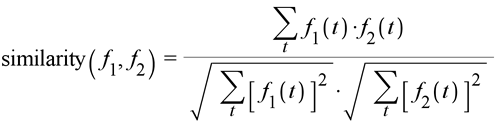## Day 1, Problem 1: Spam

Unsolicited email (spam) is annoying and clutters your mailbox. You are to write a spam filter - a program that reads email messages of regular ASCII characters and tries to determine whether or not each message is spam.

How can we determine whether or not a message is spam? Spam contains words and phrases that are not common in genuine email messages. For example, the phrase

MAKE MONEY FAST, HONEY!!

is in all-uppercase, contains the word "money" and ends with a double exclamation mark.

One way to create a spam filter is to read through many spam and non-spam messages and to come up with a set of rules that will classify any particular message as spam or not. This process can be tedious and error prone to do manually. Instead you will write a program to automate the process.

A useful step in automatic classification is to split the text up into set of trigrams. A trigram is a sequence of three adjacent characters that appear in the message. A trigram is case sensitive. The example above is composed of the trigrams:

MAK AKE KE E M MO MON ONE NEY EY Y F FA FAS AST ST, T, , H HO HON ONE NEY EY! Y!!

If we examine a sample of spam and non-spam messages we find that some trigrams are more common in spam; whereas others are more common in non-spam. This observation leads to a classification method:

• Examine a sample consisting of a large number of spam messages. Count the number of times that each trigram occurs. In the example above, there are 20 distinct trigrams; the trigrams ONE and NEY occur twice each and the remaining 18 trigrams occur once each. (Trigrams that do not occur are considered to occur 0 times.) More formally, for each trigram t we compute the frequency fspam(t) with which it occurs in the sample of spam.
• Examine a sample consisting of a large number of non-spam messages. Compute fnon-spam(t), the frequency with which each trigram t appears in the sample of non-spam.
• For a each message to be filtered, compute fmessage(t) for each trigram t.
• If fmessage resembles fspam more closely than it resembles fnon-spam it is determined to be spam; otherwise it is determined to be non-spam.
• A similarity measure determines how closely f1 and f2 resemble one another. One of the simplest measures is the cosine measure:Then we say that a message is spam if

similarity(fmessage, fspam) > similarity(fmessage, fnon-spam)

### Input

The first line of input contains three integers: s the number of sample spam messages to follow; n the number of sample non-spam messages to follow; c the number of messages to be classified as spam or non-spam, based on trigram the trigram frequencies of the sample messages. Each message consists of several lines of text and is terminated by a line containing "ENDMESSAGE". This line will not appear elsewhere in the input, and is not considered part of the message.

### Output

For each of the c messages, your program will output two lines. On the first line, output similarity(fmessage, fspam) and similarity(fmessage, fnon-spam). On the second line print the classification of the message ("spam" or "non-spam"). Round the numbers to five decimal digits.

When forming trigrams, we never include a newline character. We don't include trigrams that span multiple lines, either. So in the first spam message of Sample Input 1, the only trigrams are:

"AAA", "BBB", "BB ", "B ", " C", " CC", and "CCC".

### Sample Input 1

2 1 1
AAAA
BBBB  CCCC
ENDMESSAGE
BBBB
ENDMESSAGE
AAAABBBB
ENDMESSAGE
AAABB
ENDMESSAGE


### Sample Output 1

0.21822 0.73030 non-spam

### Sample Input 2

Found in this file

### Sample Output 2

0.28761 0.20595 spam 0.44314 0.49243non-spam

Point Value: 10
Time Limit: 3.00s
Memory Limit: 16M

Languages Allowed:
C++03, PAS, C, HASK, ASM, RUBY, PYTH2, JAVA, PHP, SCM, CAML, PERL, C#, C++11, PYTH3

• (0/0)
On the "spam" component on the last test case only. Can anyone suggest a reason why? Thanks,

• (0/0)
The lengths of messages will not exceed ~5000 characters. In addition, 1 <= s, n, c <= 5.

• (0/2)
... how the cosine measure works?

• (3/0)
Let (x0, x1, x2, x3, ...) be the trigraph frequencies for X, and (y0, y1, y2, y3, ...) be the trigraph frequencies for Y. Then, the similarity between X and Y is given by
(x0*y0 + x1*y1 + x2*y2 + x3*y3 + ...) / (sqrt(x0^2 + x1^2 + x2^2 + x3^2 + ...) sqrt(y0^2 + y1^2 + y2^2 + y3^2 + ...))

Essentially, if X and Y are vectors to the points (x0, x1, x2, x3, ...) and (y0, y1, y2, y3, ...) in N-dimensional space, then the cosine measure gives the cosine of the angle between these two vectors. Since the cosine is monotone decreasing on [0,pi], the higher the cosine measure, the smaller the angle (that is, the closer are the vectors X and Y). It also follows that the cosine measure has an absolute value less than or equal to one.